Gamedev Framework (gf)  0.5.0 A C++11 framework for 2D games
gf::Ease Class Reference

Predefined easing functions. More...

#include <gf/Easings.h>

## Public Member Functions

Ease ()=delete
Deleted constructor. More...

## Static Public Member Functions

static float linear (float t)
Linear easing. More...

static float smooth (float t)
Smooth easing. More...

static float smoother (float t)
Smoother easing. More...

static float quadIn (float t)
Ease-In flavor of quad easing. More...

static float quadOut (float t)
Ease-Out flavor of quad easing. More...

static float quadInOut (float t)
Ease-In-Out flavor of quad easing. More...

static float quadOutIn (float t)
Ease-Out-In flavor of quad easing. More...

static float cubicIn (float t)
Ease-In flavor of cubic easing. More...

static float cubicOut (float t)
Ease-Out flavor of cubic easing. More...

static float cubicInOut (float t)
Ease-In-Out flavor of cubic easing. More...

static float cubicOutIn (float t)
Ease-Out-In flavor of cubic easing. More...

static float quartIn (float t)
Ease-In flavor of quart easing. More...

static float quartOut (float t)
Ease-Out flavor of quart easing. More...

static float quartInOut (float t)
Ease-In-Out flavor of quart easing. More...

static float quartOutIn (float t)
Ease-Out-In flavor of quart easing. More...

static float quintIn (float t)
Ease-In flavor of quint easing. More...

static float quintOut (float t)
Ease-Out flavor of quint easing. More...

static float quintInOut (float t)
Ease-In-Out flavor of quint easing. More...

static float quintOutIn (float t)
Ease-Out-In flavor of quint easing. More...

static float circIn (float t)
Ease-In flavor of circ easing. More...

static float circOut (float t)
Ease-Out flavor of circ easing. More...

static float circInOut (float t)
Ease-In-Out flavor of circ easing. More...

static float circOutIn (float t)
Ease-Out-In flavor of circ easing. More...

static float sineIn (float t)
Ease-In flavor of sine easing. More...

static float sineOut (float t)
Ease-Out flavor of sine easing. More...

static float sineInOut (float t)
Ease-In-Out flavor of sine easing. More...

static float sineOutIn (float t)
Ease-Out-In flavor of sine easing. More...

static float backIn (float t)
Ease-In flavor of back easing. More...

static float backOut (float t)
Ease-Out flavor of back easing. More...

static float backInOut (float t)
Ease-In-Out flavor of back easing. More...

static float backOutIn (float t)
Ease-Out-In flavor of back easing. More...

static float bounceIn (float t)
Ease-In flavor of bounce easing. More...

static float bounceOut (float t)
Ease-Out flavor of bounce easing. More...

static float bounceInOut (float t)
Ease-Out-In flavor of bounce easing. More...

static float bounceOutIn (float t)
Ease-In-Out flavor of bounce easing. More...

static float elasticIn (float t)
Ease-In flavor of elastic easing. More...

static float elasticOut (float t)
Ease-Out flavor of elastic easing. More...

static float elasticInOut (float t)
Ease-In-Out flavor of elastic easing. More...

static float elasticOutIn (float t)
Ease-Out-In flavor of elastic easing. More...

static float expoIn (float t)
Ease-In flavor of expo easing. More...

static float expoOut (float t)
Ease-Out flavor of expo easing. More...

static float expoInOut (float t)
Ease-In-Out flavor of expo easing. More...

static float expoOutIn (float t)
Ease-Out-In flavor of expo easing. More...

## Detailed Description

Predefined easing functions.

This class defines usual easing functions.

# First set of easing functions

The first set of functions are common interpolation functions.

• linear

$f(t) = t$

• smooth

$f(t) = -2 * t^3 + 3 * t^2$

• smoother

$f(t) = 6 * t^5 - 15 * t^4 + 10 * t^3$

Here are the plots of these functions:

Functions Plot
linearsmooth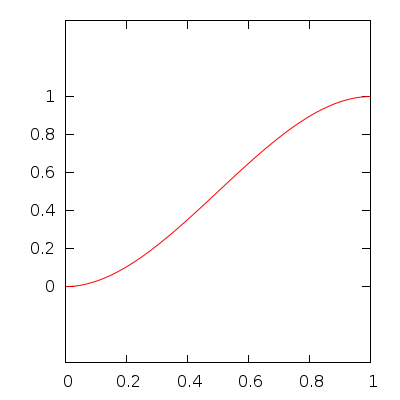smoother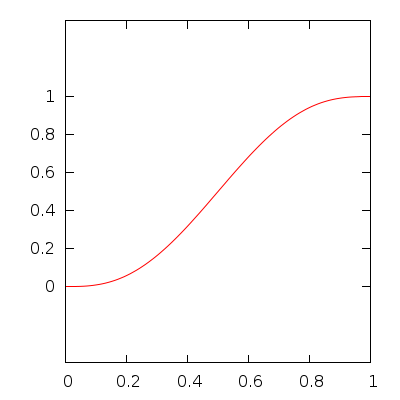# Second set of easing functions

## Definitions

The second set are the easing functions defined by Robert Penner. From a base function $$f(t)$$, four flavors are defined:

• Ease-In: start slow and speed up at the end

$\mathtt{easeIn}(t) = f(t)$

• Ease-Out: start fast and slow down at the end

$\mathtt{easeOut}(t) = 1 - \mathtt{easeIn}(1 - t)$

• Ease-In-Out: start slow, then speed up in the middle and finally slow down at the end

$\mathtt{easeInOut}(t) = \begin{cases} \mathtt{easeIn}(2 \times t) \div 2 & \text{if } t < 0.5 \\ \mathtt{easeOut}(2 \times t + 1) \div 2 + 0.5 & \text{if } t \geq 0.5 \end{cases}$

• Ease-Out-In: start fast, then slow down in the middle and finally speed up at the end

$\mathtt{easeOutIn}(t) = \begin{cases} \mathtt{easeOut}(2 \times t) \div 2 & \text{if } t < 0.5 \\ \mathtt{easeIn}(2 \times t + 1) \div 2 + 0.5 & \text{if } t \geq 0.5 \end{cases}$

## Base functions

Here are the base functions:

• quad

$f(t) = t^2$

• cubic

$f(t) = t^3$

• quart

$f(t) = t^4$

• quint

$f(t) = t^5$

• sine

$f(t) = 1 - \cos\left(t \times \frac{\pi}{2}\right)$

• expo

$f(t) = \begin{cases} 0 & \text{if } t = 0 \\ 2^{10 \times (t - 1)} & \text{if } t > 0 \end{cases}$

• circ

$f(t) = 1 - \sqrt{1 - t^2}$

• back

$f(t) = t^2 \times (2.70158 \times t - 1.70158)$

• bounce

$f(t) = \begin{cases} 1 - 7.5625 \times (1 - t)^2 & \text{if } 1 - t < 1 / 2.75 \\ 1 - (7.5625 \times (1 - t - 1.5 / 2.75)^2 + 0.75) & \text{if } 1 - t < 2 / 2.75 \\ 1 - (7.5625 \times (1 - t - 2.25 / 2.75)^2 + 0.9375) & \text{if } 1 - t < 2.5 / 2.75 \\ 1 - (7.5625 \times (1 - t - 2.625 / 2.75)^2 + 0.984375) & \text{otherwise} \end{cases}$

• elastic

$f(t) = -2^{10 \times (t - 1)} \times \sin\left(\left((t - 1) - \frac{0.3}{4}\right) \times \frac{2 \times \pi}{0.3}\right)$

## Plots

Here are the plots for all the defined easing functions:

Function Ease-In Ease-Out Ease-In-Out Ease-Out-In
quad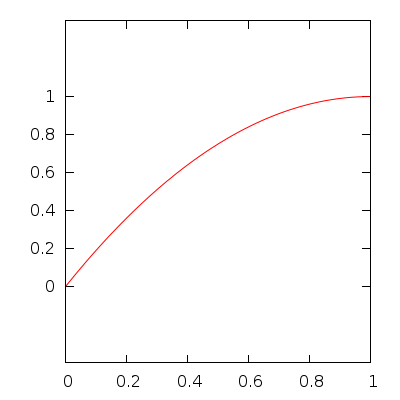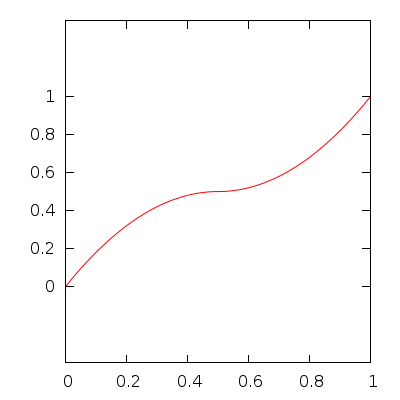cubic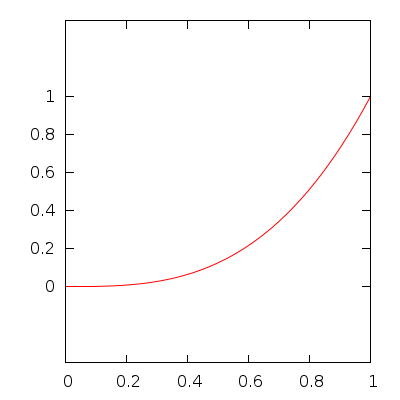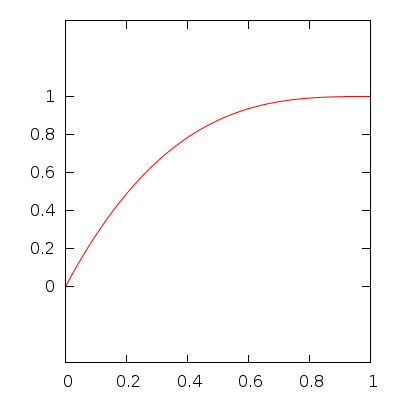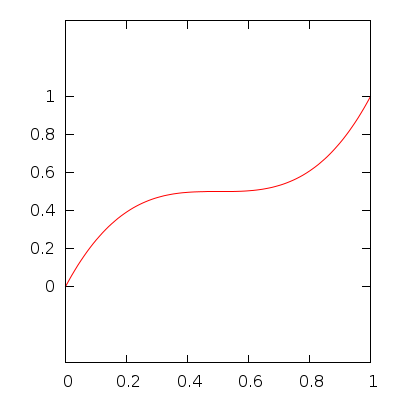quart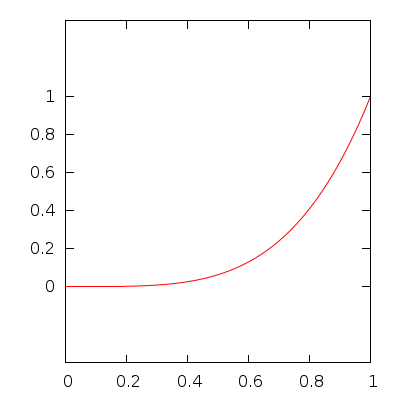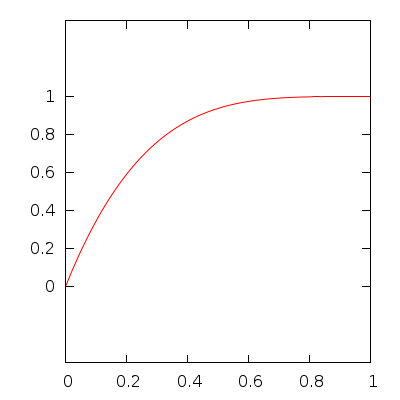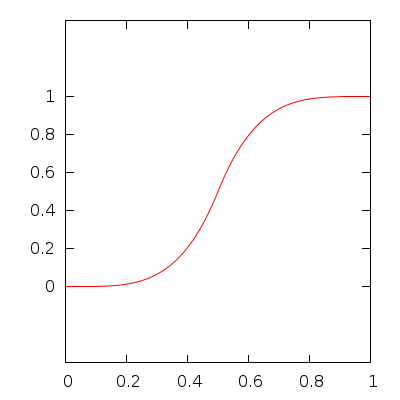quint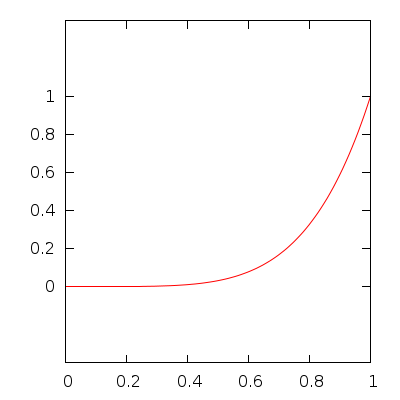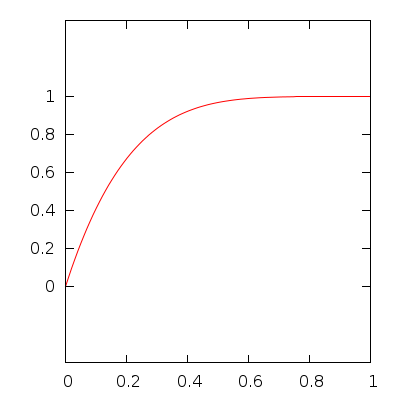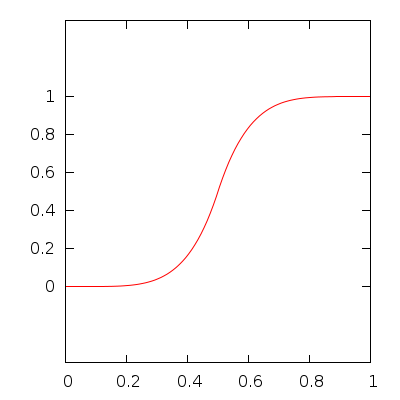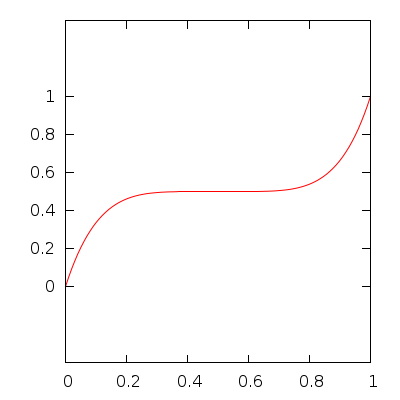sine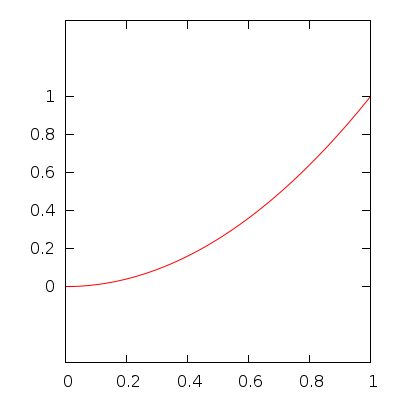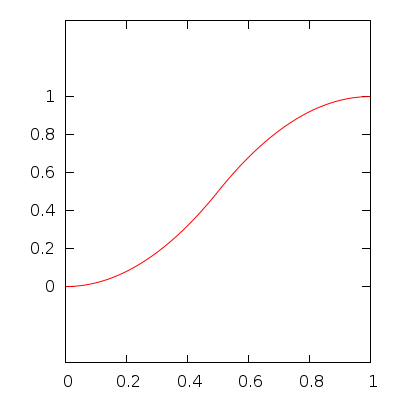expo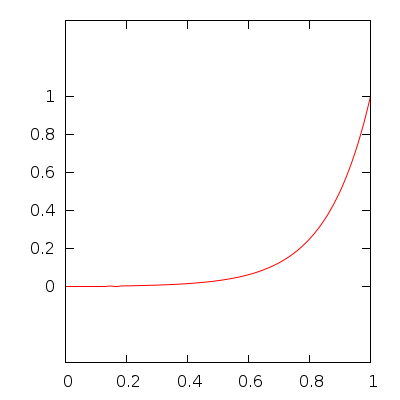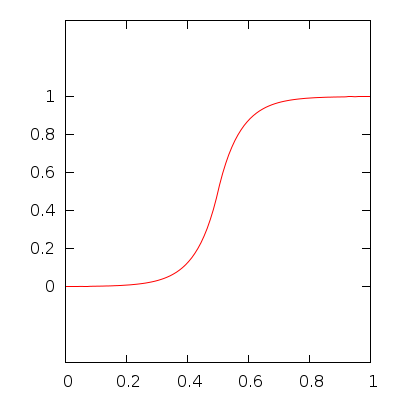circ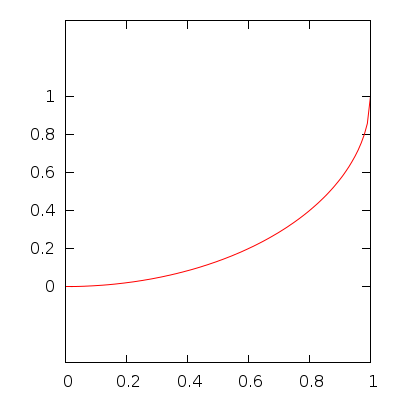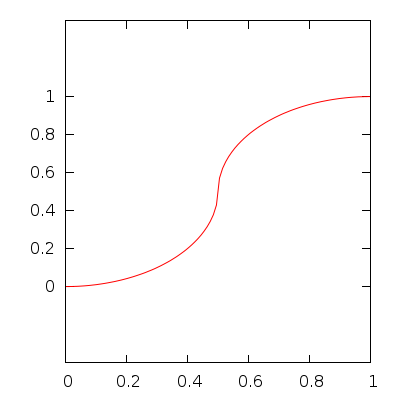back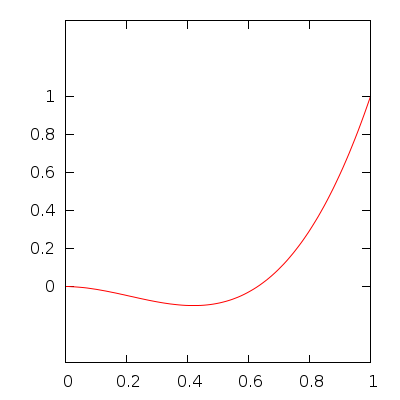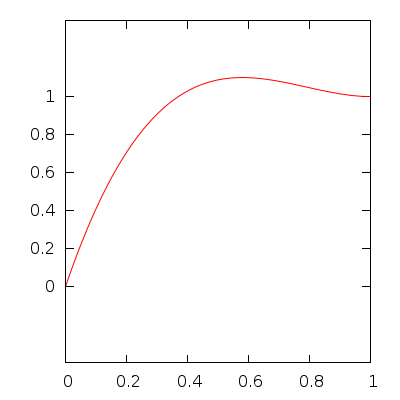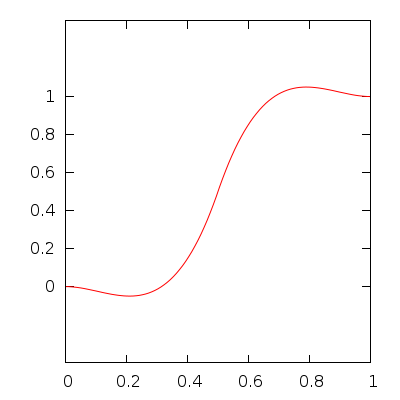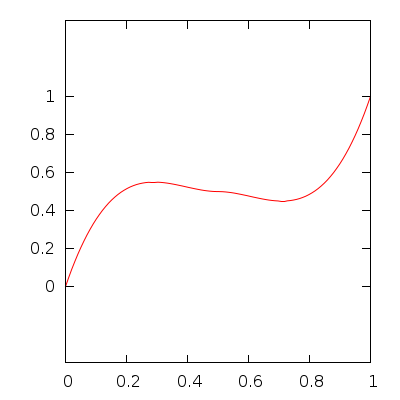bounce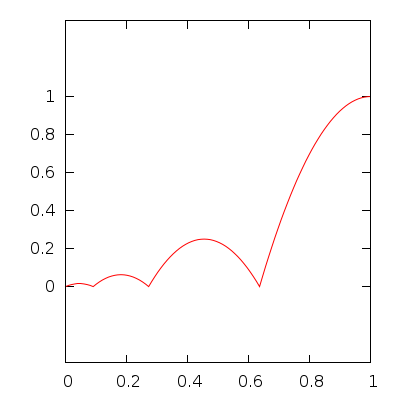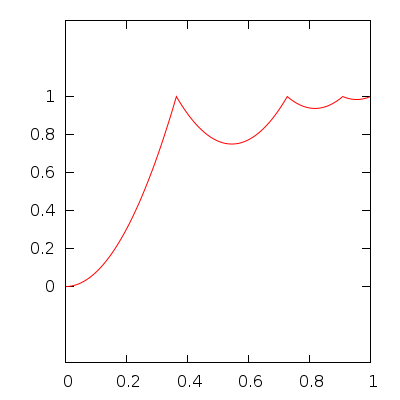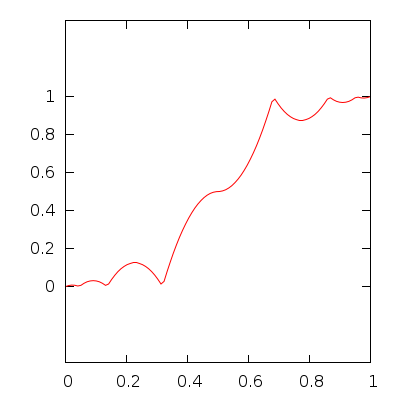elastic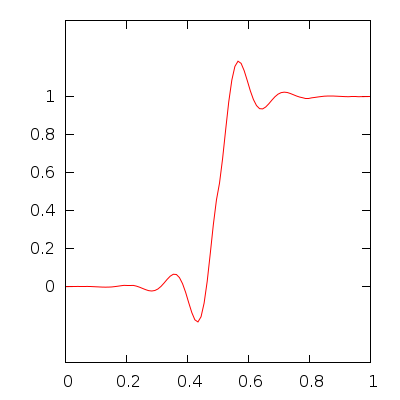See also
gf::Easing
Robert Penner's Easing Functions
Easing Functions Cheat Sheet

## ◆ Ease()

 gf::Ease::Ease ( )
delete

Deleted constructor.

## ◆ backIn()

 static float gf::Ease::backIn ( float t )
static

Ease-In flavor of back easing.

## ◆ backInOut()

 static float gf::Ease::backInOut ( float t )
static

Ease-In-Out flavor of back easing.

## ◆ backOut()

 static float gf::Ease::backOut ( float t )
static

Ease-Out flavor of back easing.

## ◆ backOutIn()

 static float gf::Ease::backOutIn ( float t )
static

Ease-Out-In flavor of back easing.

## ◆ bounceIn()

 static float gf::Ease::bounceIn ( float t )
static

Ease-In flavor of bounce easing.

## ◆ bounceInOut()

 static float gf::Ease::bounceInOut ( float t )
static

Ease-Out-In flavor of bounce easing.

## ◆ bounceOut()

 static float gf::Ease::bounceOut ( float t )
static

Ease-Out flavor of bounce easing.

## ◆ bounceOutIn()

 static float gf::Ease::bounceOutIn ( float t )
static

Ease-In-Out flavor of bounce easing.

## ◆ circIn()

 static float gf::Ease::circIn ( float t )
static

Ease-In flavor of circ easing.

## ◆ circInOut()

 static float gf::Ease::circInOut ( float t )
static

Ease-In-Out flavor of circ easing.

## ◆ circOut()

 static float gf::Ease::circOut ( float t )
static

Ease-Out flavor of circ easing.

## ◆ circOutIn()

 static float gf::Ease::circOutIn ( float t )
static

Ease-Out-In flavor of circ easing.

## ◆ cubicIn()

 static float gf::Ease::cubicIn ( float t )
static

Ease-In flavor of cubic easing.

## ◆ cubicInOut()

 static float gf::Ease::cubicInOut ( float t )
static

Ease-In-Out flavor of cubic easing.

## ◆ cubicOut()

 static float gf::Ease::cubicOut ( float t )
static

Ease-Out flavor of cubic easing.

## ◆ cubicOutIn()

 static float gf::Ease::cubicOutIn ( float t )
static

Ease-Out-In flavor of cubic easing.

## ◆ elasticIn()

 static float gf::Ease::elasticIn ( float t )
static

Ease-In flavor of elastic easing.

## ◆ elasticInOut()

 static float gf::Ease::elasticInOut ( float t )
static

Ease-In-Out flavor of elastic easing.

## ◆ elasticOut()

 static float gf::Ease::elasticOut ( float t )
static

Ease-Out flavor of elastic easing.

## ◆ elasticOutIn()

 static float gf::Ease::elasticOutIn ( float t )
static

Ease-Out-In flavor of elastic easing.

## ◆ expoIn()

 static float gf::Ease::expoIn ( float t )
static

Ease-In flavor of expo easing.

## ◆ expoInOut()

 static float gf::Ease::expoInOut ( float t )
static

Ease-In-Out flavor of expo easing.

## ◆ expoOut()

 static float gf::Ease::expoOut ( float t )
static

Ease-Out flavor of expo easing.

## ◆ expoOutIn()

 static float gf::Ease::expoOutIn ( float t )
static

Ease-Out-In flavor of expo easing.

## ◆ linear()

 static float gf::Ease::linear ( float t )
static

Linear easing.

This is the default easing function

## ◆ quadIn()

 static float gf::Ease::quadIn ( float t )
static

Ease-In flavor of quad easing.

## ◆ quadInOut()

 static float gf::Ease::quadInOut ( float t )
static

Ease-In-Out flavor of quad easing.

## ◆ quadOut()

 static float gf::Ease::quadOut ( float t )
static

Ease-Out flavor of quad easing.

## ◆ quadOutIn()

 static float gf::Ease::quadOutIn ( float t )
static

Ease-Out-In flavor of quad easing.

## ◆ quartIn()

 static float gf::Ease::quartIn ( float t )
static

Ease-In flavor of quart easing.

## ◆ quartInOut()

 static float gf::Ease::quartInOut ( float t )
static

Ease-In-Out flavor of quart easing.

## ◆ quartOut()

 static float gf::Ease::quartOut ( float t )
static

Ease-Out flavor of quart easing.

## ◆ quartOutIn()

 static float gf::Ease::quartOutIn ( float t )
static

Ease-Out-In flavor of quart easing.

## ◆ quintIn()

 static float gf::Ease::quintIn ( float t )
static

Ease-In flavor of quint easing.

## ◆ quintInOut()

 static float gf::Ease::quintInOut ( float t )
static

Ease-In-Out flavor of quint easing.

## ◆ quintOut()

 static float gf::Ease::quintOut ( float t )
static

Ease-Out flavor of quint easing.

## ◆ quintOutIn()

 static float gf::Ease::quintOutIn ( float t )
static

Ease-Out-In flavor of quint easing.

## ◆ sineIn()

 static float gf::Ease::sineIn ( float t )
static

Ease-In flavor of sine easing.

## ◆ sineInOut()

 static float gf::Ease::sineInOut ( float t )
static

Ease-In-Out flavor of sine easing.

## ◆ sineOut()

 static float gf::Ease::sineOut ( float t )
static

Ease-Out flavor of sine easing.

## ◆ sineOutIn()

 static float gf::Ease::sineOutIn ( float t )
static

Ease-Out-In flavor of sine easing.

## ◆ smooth()

 static float gf::Ease::smooth ( float t )
static

Smooth easing.

See also
Smoothstep - Wikipedia

## ◆ smoother()

 static float gf::Ease::smoother ( float t )
static

Smoother easing.

See also
Smootherstep - Wikipedia